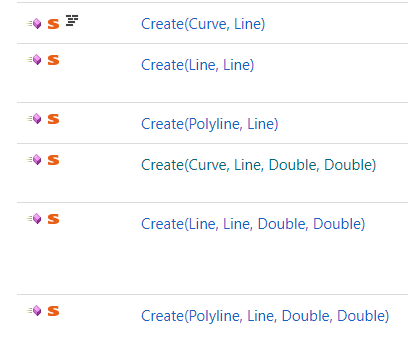# Rhino Common RevSurface.Create not accepting NURBS curve

I’m currently trying to create a revolved surface from a NURBS curve.

There should be quite a few methods of adding a surface of revolution using RhinoCommon, I’m currently trying to utilize this one:

https://developer.rhino3d.com/api/RhinoCommon/html/M_Rhino_Geometry_RevSurface_Create_1.htm

But it won’t let me:

`Message: expected Polyline, got NurbsCurve`

I know there is also a polyline variant of this function, but shouldn’t it be able to deal with both:A `Polyline` isn’t a curve. Rather, it’s just an array of points.

Use a `PolylineCurve` instead. You can create them from a `Polyline`.

https://developer.rhino3d.com/5/api/RhinoCommon/html/T_Rhino_Geometry_PolylineCurve.htm

– Dale

Unfortunately the curve I’m trying to revolve isn’t suited to be converted to a polyline, since the line segments aren’t straight. So I do in fact have to revolve the NURBS curve. Should’nt the `Create(Curve, Line, Double, Double)` be able to do exactly this?

Edit: I do realize that there is a difference between the NurbsCurve and the Curve method and that perhaps this is where it all goes wrong, but could I then convert my nurbs curve to curve?

Anyone?

By testing RevSurface.Create in Python, I found that the error reported for a type mismatch is sometimes misleading. Some examples:

RevSurface.Create(a, b) : `Message: expected Line, got _`,
when b is a Line, but a is not a Curve.

RevSurface.Create(a, b) : `Message: expected Line, got _Curve`,
when a is a _Curve, but b is not a Line.

RevSurface.Create(a, b, c, d) : `Message: expected Polyline, got _Curve`,
when an is a _Curve but b is not a Line.

So, `Message: expected Polyline, got NurbsCurve` would be stated even if you are attempting to pass, for example, a GUID as the Line parameter.

This seems to work:

``````import System
import Rhino
import scriptcontext as sc

p0 = Rhino.Geometry.Point3d(0,0,0)
p1 = Rhino.Geometry.Point3d(0,0,1)
p2 = Rhino.Geometry.Point3d(10,0,5)
p3 = Rhino.Geometry.Point3d(5,0,10)
axis = Rhino.Geometry.Line(p0, p1)
a0 = 0
a1 = 2.0 * System.Math.PI

# Create from Line, Line
line = Rhino.Geometry.Line(p0, p2)
srf0 = Rhino.Geometry.RevSurface.Create(line, axis, a0, a1)

# Create from Curve, Line
crv = Rhino.Geometry.LineCurve(p0, p3)
srf1 = Rhino.Geometry.RevSurface.Create(crv, axis, a0, a1)

# Create from Polyline, Line
pline = Rhino.Geometry.Polyline()
srf2 = Rhino.Geometry.RevSurface.Create(pline, axis, a0, a1)

sc.doc.Views.Redraw()
``````

What am I missing?

– Dale

This seems to be true. For example my case is the third one. However, I am not able to use a `Line` for my axis. It returns an error stating `expected Vector3d, got Line`.

What you’re currently missing is the fact that I’m not using either a line curve or a polyline curve. I’m trying to use a NURBS Curve. This should be possible according to the documentation, but it is not.

I’ve created a workaround using `SweepOneRail` though:

``````def revolveBaseCurves(geometry,N):
path = Rhino.Geometry.NurbsCurve.CreateFromArc(arc)
sweepClass = Rhino.Geometry.SweepOneRail()
for curve in geometry:
brep = Rhino.Geometry.SweepOneRail.PerformSweep(sweepClass,path,curve)
``````

Here `O()` is the Point3d of the origin and `N` determines the arc length (`N=1` would result in a full rotation). `N` is specific to my problem, but you could just as well derive your own rotation in radians.

This seems to work:

``````import System
import Rhino
import scriptcontext as sc

pt_start = Rhino.Geometry.Point3d(5,0,0)
pt_interior = Rhino.Geometry.Point3d(8,0,10)
pt_end = Rhino.Geometry.Point3d(15,0,15)
arc = Rhino.Geometry.Arc(pt_start, pt_interior, pt_end)
nc = arc.ToNurbsCurve() # <- NURBS

pt_from = Rhino.Geometry.Point3d(0,0,0)
pt_to = Rhino.Geometry.Point3d(0,0,1)
line = Rhino.Geometry.Line(pt_from, pt_to)

ang_start = 0
ang_end = 2.0 * System.Math.PI
srf = Rhino.Geometry.RevSurface.Create(nc, line, ang_start, ang_end)